# Insights into Easier Trigonometry

## Trigonometry becomes easier when one can relate the isentities to what is known. In the current world where online classes are more common it is important to understand.

Often Mathematics books are just a little vague as happens when something is so clear to the author that the author has no idea an explanation is needed. In other cases things are stated once, and if you do not pay attention to the details you can later find yourself asking how did we get to this point later. Trigonometry has several simple ideas that can be daunting if we just miss those few words, or made simple if we just understand a little deeper.

Another problem occurs in all of mathematics. Definitions are often wordy, and one might wonder why all the extra words that seem to be there just to clutter the idea being presented. Unfortunately, those words are usually needed, albeit for cases that might not occur at the level the material is intended.

The solution is a few clarifying words can be added to help make things understandable.

### Trigonometry Is Based on a Unit Circle

##### But Any Circle Will Do

We are told the basic trigonometric functions are based on a unit circle, but then angles not associated with a unit circle are used freely. If we try to make a right triangle with a hypotenuse other than one the triangle simply does not fit into the unit circle. So, why do the ratios of the sides of any right triangle work, whether we are using a right triangle with a hypotenuse of one, or some other hypotenuse? This is simple to understand. If we extend or contract a right triangle with a unit hypotenuse to any size, we still have a right triangle. And the angle with which we are concerned still has the same measure. Well, if two pairs of angles of two triangles have the same measures as their corresponding angle, so must the third pair of angles match in measure. Hence, we have similar triangles, so the ratio of corresponding sides should be the same value. Even though a ratio of two sides of the unit triangle are used to define a basic trigonometric identities, it is no deep mystery why we are not constrained to the unit circle. Any right triangle has the trigonometric identities found in the same manner as those of a unit triangle because t is a similar triangle to a unit triangle having the same angles.

### How Do We Get the Pythagorean Identities?

##### Think of the Pythagorean Theorem

Well, take a right triangle with the vertex of one of its angles at the origin, and one of its sides lying along the x-axis with length x. The hypotenuse goes from the origin to the point (x,y), which is y units above (x, 0). So the three sides are xy, and r in length. For the unit circle r is 1. Since we have a right triangle the Pythagorean Theorem holds, x2 + y2 = r2. If you divide through by r2 you get (x/r)2 + (y/r)2 = 1. But x/r is cos(A) and y/r is sin(A). Hence cos2(A) + sin2(A) = 1, which is often seen as sin2(A) + cos2(A) = 1. To get tan2(A) + 1 = sec2(A) or 1 + cot2(A) = csc2(A) divide the first Pythagorean identity by sin2(A) and by cos2(A), respectively.

When either x or y is zero in length we no longer have a triangle. Recall that you are never allowed to divide by zero, so words must be added for these special cases, but this is much easier to explain without cumbersome mathematics.

### Pythagorean Theorem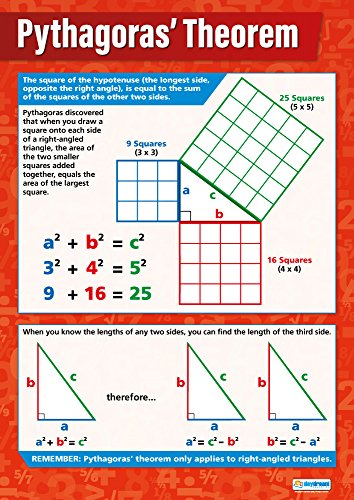Pythagoras' Theorem Math Poster – Glo...View on Amazon

### What Exactly Is a Co-Function?

##### Think of Complementry Angles

It is often said that the sine and the cosine are related, but how? Well, the prefix “co” is short for complementary, as in two angles are complementary if their measures add to ninety degrees. In a right triangle, the two angles other than the right angle must add to ninety degrees in measure. So, the cosine of an angle is the sine of the complementary angle, and likewise with tangent and cotangent, and with secant and cosecant. Notice the adjacent side becomes the opposite side for the complementary angle, and the opposite side becomes the adjacent side.

### Is There an Easy Way to Know in What Quadrants the Trigonometric Functions Are Positive Or Negative?

##### Think of the Coordinate System

First, we must be flexible with the use of triangles. When x or y is negative we can still use a triangle to get the magnitude of the sides, but we must allow for negative values in the ratios of the sides. Here, triangles are a tool, but we must think about whether x and y are positive or negative.

The memory aids can easily be forgotten. To determine the sign of a trigonometric function, just think of the ratio for the function in terms of xy, and r. If r must always be positive, then just think about in which quadrants x and y are positive, and in which quadrants they are negative. Make your ratio and remember that if both signs are alike the ratio is positive, and if the signs differ the ratio is negative.

### What Are the Quotient and Reciprocal Identies?

The reciprocal identies are by looking at the ratios that form the six trigonometric functions and observing that each function has a reciprocal, provided no division by zero occurs. These are sin(A) = 1/csc(A), cos(A) = i/sec(A), and tan(A) = 1/cot(A). The reciprocals of the above work, giving the six reciprocal identies.

The quotient identities are tan(A) = sin(A)/cos(A), which comes from simplifying y/x = (y/r)/(x/r) by cancelling out the r. The other quotient identity, cot(A) = cos(A)/sin(A) is explained similarly. Notice this is not dependent upon which quadrant one is in, as long as no division by zero occurs.

### How Do You Remember the Values of the Trigonometric Functions at Thirty, Forty-Five, and Sixty Degrees?

Look at the pattern for the sine function. If you think of the 1/2 for thirty degrees, it can also be thought of as the square root of 1 over 2. Now look at all three values in order, and notice the pattern. The square root of one, two, and three, in order, always divided by two.

For the cosine, just go backwards. This is because “co” means complementary angle, so the cosine of thirty degrees is the sine of sixty degrees. As for the tangent and cotangent, use the quotient identities. The secant and cosecant use the reciprocal identities.

### Trigonometry Chart

##### Unit Circle and Special Angles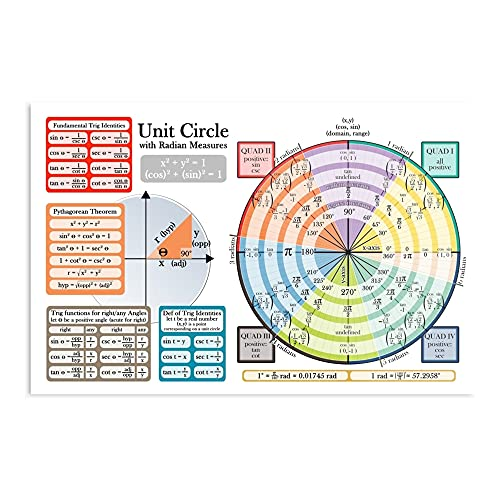kineticards Circle Unit College Trigonometry University Geometry Ma...View on Amazon

### More Trigonometry

Remember the unit crcle is the basis of trigonometry. Not every problem with angles involves a right triangle. One can use right triangles to go beyond the basics, and certainly those right triangles are part of the proofs for more complex identies. But we should avail ourselfes to the work done by others and simply accept such things as the Law of Sines and the Law of Cosines, the trignonmetric identities finvolving the sum and the difference of angles, and so on. In some cases there is a need to not only know if something is positive or negative, but whether the numerator or the denominator is itself positive or negative. In trigonometry it is not always enough to know that 1/2 is positive, we must know if it came from 1/2 or -1/(-2).

### Law of Sines and Law of Cosines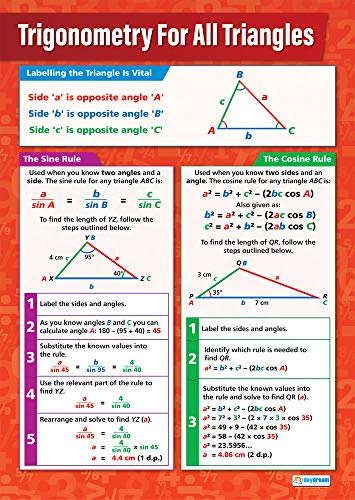Trigonometry for All Triangles Math Poster – Laminated – 33” x 23.5...View on Amazon

This article contains links to affiliate programs and Adsense advertising.  These must use cookies to allow for proper crediting. As a Viglink Associate and Amazon Associate I earn from qualifying purchases.

Updated: 08/09/2022, blackspanielgallery
Thank you! Would you like to post a comment now?
1

Only logged-in users are allowed to comment. Login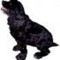blackspanielgallery 23 days ago

The "co" stands for complementary, meaning the angle that is added to obtain 90 degrees. All "co" functions look like the functions, but the values for 30 degrees for one are the values for 60 degrees for the other. In other words, tan(45 deg) = cot (40 deg), tan 30 deg) -= cot 60 deg), and so on. The same is true for sine and cosine, as well as secant and cosecant.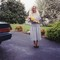DerdriuMarriner 23 days ago

blackspanielgallery, Thank you for practical information, pretty pictures and product lines.

In particular I appreciate the examples that always anchor your explanations.

Are the attractive charts that you offer the only assists? Or, for example, would there be something similar for cotangents and tangents?

#### You might also like

Buttons from the Rag Bag! Button Jar Math Activities

Save the buttons from discarded clothing and recycle them as fun, hands-on ma...

Kumon Math Review

A mother's review of the Kumon Math program including suggestions for kumon a...

Disclosure: This page generates income for authors based on affiliate relationships with our partners, including Amazon, Google and others.